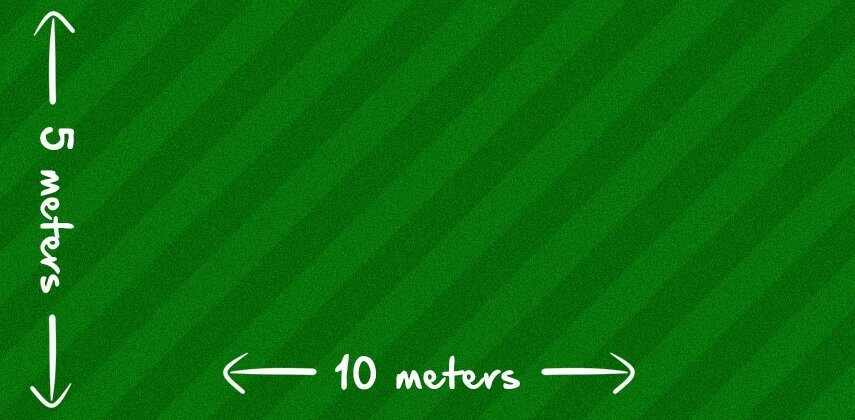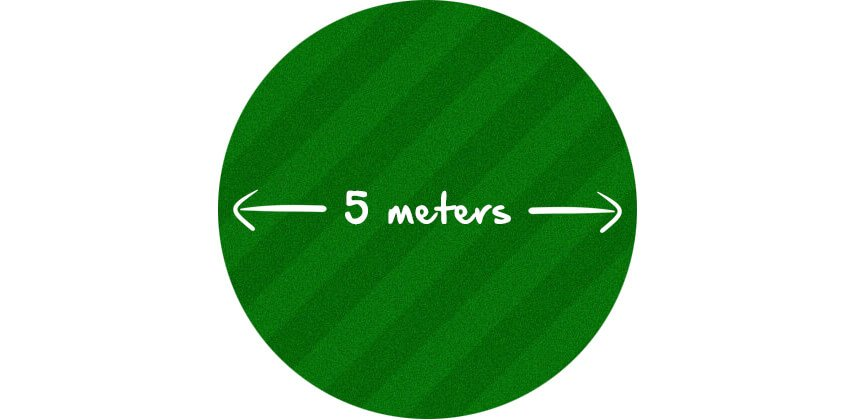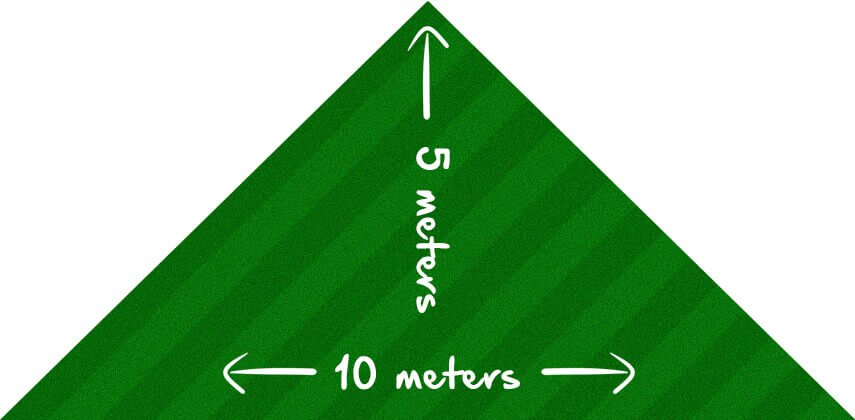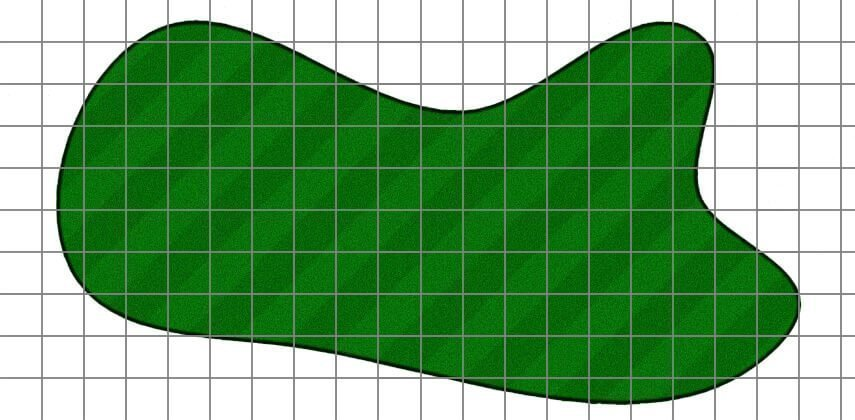# Grass Seed Calculator: How Much Grass Seed Do I Need?

Contents

If you’re sowing a new lawn from scratch or overseeding an existing lawn, as part of your lawn care regime, you’ve probably asked yourself, ‘How Much Grass Seed Do I Need?’

## Work Out How Much You Need With Our Grass Seed Calculator

This grass seed calculator will work out how much seed you need, in KG, for sowing a new lawn or overseeding an existing one.

2. Enter the dimensions of your lawn
3. The calculator will then tell you how much grass seed you need

[CP_CALCULATED_FIELDS id=”8″]

## How to Manually Calculate How Much Grass Seed You Need

Do you prefer to manually work out how much grass seed you need? Then I’ll show you how to do in a moment firstly, remember these numbers.

If you are sowing a new lawn from scratch, spread your grass seed at a rate of 35g per square meter.

For overseeding an existing lawn, spread your seed at 25g per square meter.

### How to Work Out How Much Seed You Need For a Square or Rectangle LawnArea = length x width

Working out the area of a square or rectangular lawn is dead simple.

Simply multiply the length by the width.

In the example above, the lawn is 10 meters long by 5 meters wide. So the maths looks like this;

10 x 5 = 50

If you’re sowing a new lawn, times the area of your lawn by the recommended application rate of 35 grams per m2.

50 (the area of your lawn) x 35 (the recommended application rate for sowing a new lawn) = 1,750 grams of grass seed.

For overseeding, just change the application rate to 25 grams:

50 (the area of your lawn) x 25 (the recommended application rate for overseeding an existing lawn) = 1,250 grams of grass seed.

### Working Out How Much Seed You Need For a Circular LawnTo measure the area of a circular lawn, take the diameter (the measurement across the circle) and divide it by 2 to get the radius which we’ll call ‘R’.

Now we need to multiply the radius by itself and then multiply that by 3.14.

The equation looks like this;

R x R x 3.14.

So if your lawn is 5 meters in diameter, this is how your work out the area;

5m (diameter) / 2 = 2.5 (R)

2.5 (R) x 2.5 (R) x 3.14 = 19.63

That’s 19.63 square meters (or 20sq meters for ease).

For sowing a new lawn multiply this by the recommended 35g per square meter;

20 (the area of your lawn) x 35 (the recommended application rate for sowing a new lawn) = 700 grams of grass seed.

If you’re overseeding, change the application rate to 25g per square meter;

78.5 (the area of your lawn) x 25 (the recommended application rate for overseeding an existing lawn) = 500 grams of grass seed.

### How to Work Out a Triangular LawnArea = 1/2 longest side x height

To figure out how much grass seed you need for a triangular lawn, take the longest side of the triangle and divide it by two.

Then multiply that number by the height.

In the example above, the triangle is 10 meters long and 5 meters high. So this is how you’d work it out;

10 (the longest side of the triangle) / 2 = 5 x 5 = 25.

That’s 25 square meters.

If you’re sowing a new lawn, multiply it by 35 grams per square meter;

25 (the area of your lawn) x 35 (the recommended application rate for sowing a new lawn) = 875 grams of grass seed.

For overseeding an existing lawn, multiply it by 25 grams per square meter;

25 (the area of your lawn) x 25 (the recommended application rate for overseeding an existing lawn) = 625 grams of grass seed.

## When a Grass Seed Calculator Doesn’t Work

As helpful as my grass seed calculator is, it only works if you have a square, rectangular, circular or triangular lawn.

However, most lawns don’t have a distinct shape at all, so how do you go about measuring them?

Well, although it’s tricker than punching some numbers into a calculator, you can estimate how much grass seed you need fairly accurately.

### How to Measure an Odd Shaped LawnOn a piece of graph paper, allocate one large square to a square meter.

Measure the length and width of your whole garden and draw it on your paper.

Next, measure any permanent feature like patios, sheds, walls, fences, pathways etc and draw them to scale. This will help you draw your lawn to a better scale.

Now measure the distance between the edges of your lawn from those features and mark them on your plan.

With all that done, draw the full outline of your lawn. This should now be a pretty accurate representation of its size.

Finally, count the full squares and make a note of the number. Then count the partial squares and make an educated as to how many full squares they add up to. Now add those numbers together.

This will give you a pretty accurate square meterage.

## In Conclusion

I hope you found my grass seed calculator useful and it made working out how much grass seed you need a bit easier.

And if you have an odd-shaped lawn or you prefer to calculate things for yourself, I hope my formulas and processes helped.

If you have any questions when it comes to working out how much grass seed you need, drop a comment below.1.